Chapter 3 Ratios and Rates Day 1 Ratios

• Slides: 53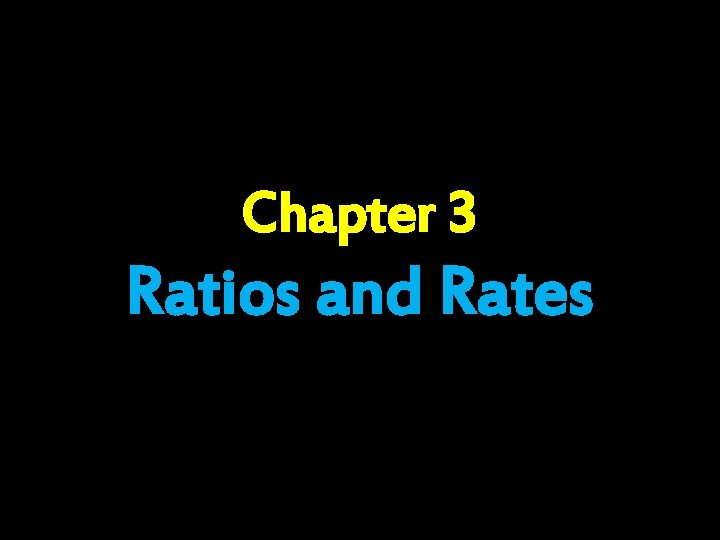Chapter 3 Ratios and Rates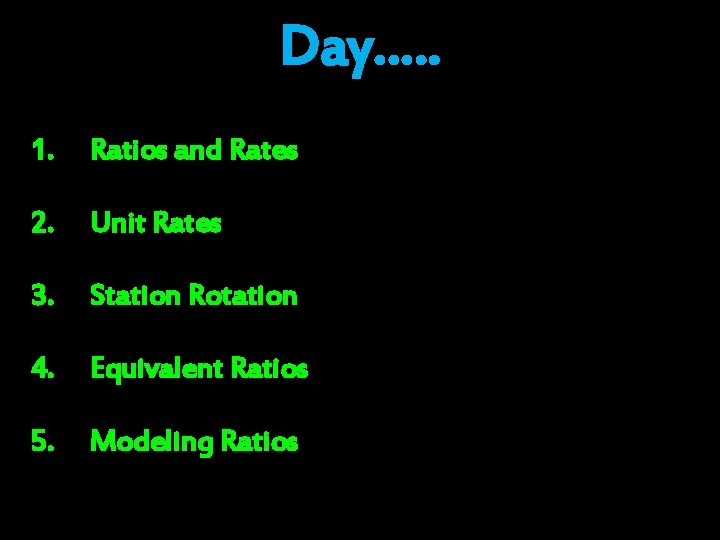Day…. . 1. Ratios and Rates 2. Unit Rates 3. Station Rotation 4. Equivalent Ratios 5. Modeling RatiosDay 1Bell Work During one year Mrs. Laymon sent 484 text messages. This number was 1/3 the number of text messages her son sent during the same year. How Many text message did her son send? Draw a diagram and justify your response. If he sent the same number of messages each month, how many messages did he send in one month?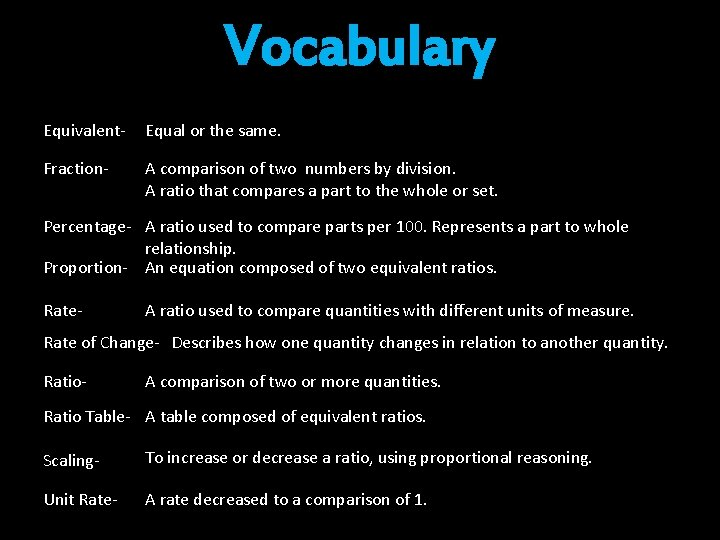Vocabulary Equivalent- Equal or the same. Fraction- A comparison of two numbers by division. A ratio that compares a part to the whole or set. Percentage- A ratio used to compare parts per 100. Represents a part to whole relationship. Proportion- An equation composed of two equivalent ratios. Rate- A ratio used to compare quantities with different units of measure. Rate of Change- Describes how one quantity changes in relation to another quantity. Ratio- A comparison of two or more quantities. Ratio Table- A table composed of equivalent ratios. Scaling- To increase or decrease a ratio, using proportional reasoning. Unit Rate- A rate decreased to a comparison of 1.I Can…. Identify and express ratios and rates.Introducing Ratios Essential Understanding: • • • A ratio is a comparison of two or more quantities. Ratios can be used to show comparisons of a… I. part to whole - (a fraction) II. whole to part - (the reciprocal of a fraction) III. part to part – I. Fraction Bar – II. Colon – III. Words – Ratios can be written in three different forms: When expressing a ratio, you must list the quantities in the order they are asked, NOT the order they are given. Example: • Ratios can be scaled to create equivalent ratios • I. Ratios are scaled down or simplified by division. I. Ratios are scaled up by multiplicationIntroducing Rates Essential Understanding: • A rate is a ratio that compares quantities with different kinds of units. Examples: A. Driving 120 miles in two hours. B. Paying \$2. 49 per gallon. C. Reading 15 pages in 20 minutes. D. Making \$15 an hour. • Unlike other ratios, the units must be included when expressing and calculating rates. Example: • Rates express the relationship between two variables. I. II. • An independent variable. A dependent variable- Rate of change is used to express the change over time between to quantities. Example:Wrap it Up • Review • Questions • Exit TicketsExit Ticket On a post it reflect on something that “Stuck with you today”.Day 2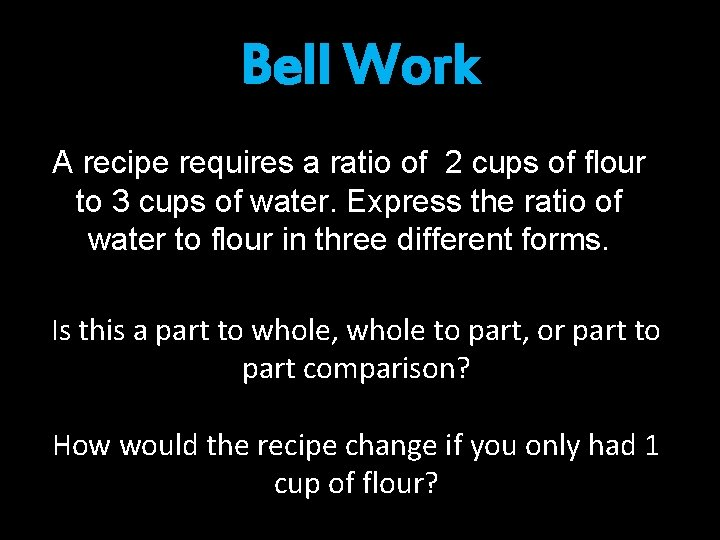Bell Work A recipe requires a ratio of 2 cups of flour to 3 cups of water. Express the ratio of water to flour in three different forms. Is this a part to whole, whole to part, or part to part comparison? How would the recipe change if you only had 1 cup of flour?Vocabulary Equivalent- Equal or the same. Fraction- A comparison of two numbers by division. A ratio that compares a part to the whole or set. Percentage- A ratio used to compare parts per 100. Represents a part to whole relationship. Proportion- An equation composed of two equivalent ratios. Rate- A ratio used to compare quantities with different units of measure. Rate of Change- Describes how one quantity changes in relation to another quantity. Ratio- A comparison of two or more quantities. Ratio Table- A table composed of equivalent ratios. Scaling- To increase or decrease a ratio, using proportional reasoning. Unit Rate- A rate decreased to a comparison of 1.Homework Check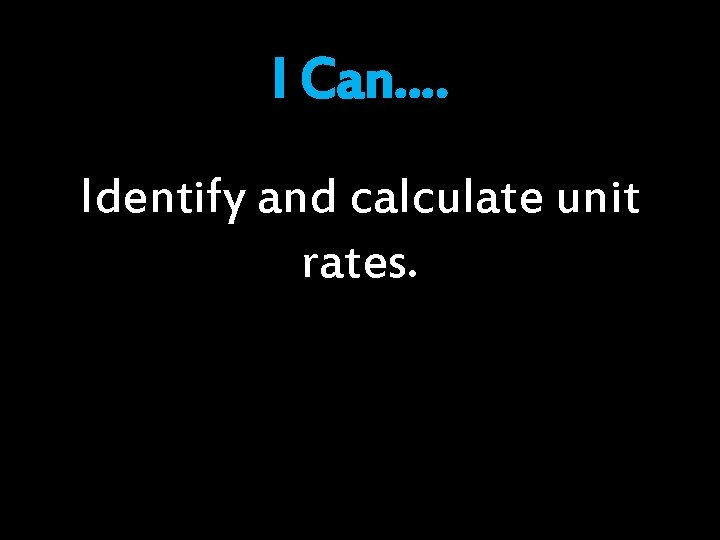I Can…. Identify and calculate unit rates.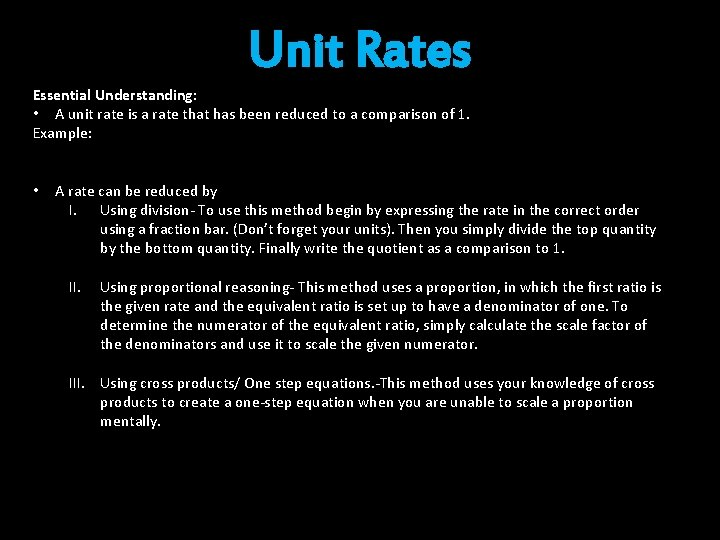Unit Rates Essential Understanding: • A unit rate is a rate that has been reduced to a comparison of 1. Example: • A rate can be reduced by I. Using division- To use this method begin by expressing the rate in the correct order using a fraction bar. (Don’t forget your units). Then you simply divide the top quantity by the bottom quantity. Finally write the quotient as a comparison to 1. II. Using proportional reasoning- This method uses a proportion, in which the first ratio is the given rate and the equivalent ratio is set up to have a denominator of one. To determine the numerator of the equivalent ratio, simply calculate the scale factor of the denominators and use it to scale the given numerator. III. Using cross products/ One step equations. -This method uses your knowledge of cross products to create a one-step equation when you are unable to scale a proportion mentally.Wrap it Up • Review • Questions • Exit Tickets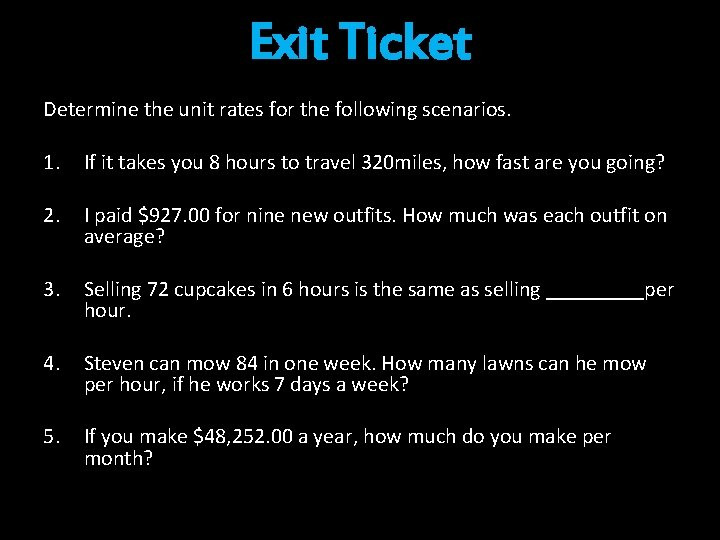Exit Ticket Determine the unit rates for the following scenarios. 1. If it takes you 8 hours to travel 320 miles, how fast are you going? 2. I paid \$927. 00 for nine new outfits. How much was each outfit on average? 3. Selling 72 cupcakes in 6 hours is the same as selling _____per hour. 4. Steven can mow 84 in one week. How many lawns can he mow per hour, if he works 7 days a week? 5. If you make \$48, 252. 00 a year, how much do you make per month?Day 3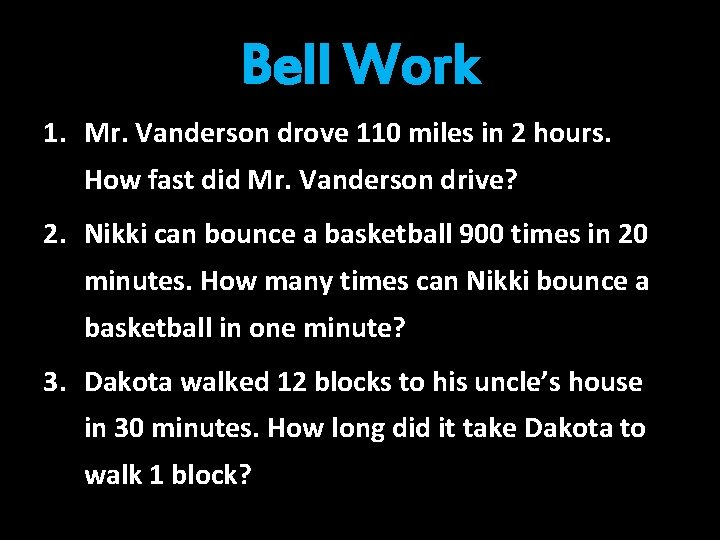Bell Work 1. Mr. Vanderson drove 110 miles in 2 hours. How fast did Mr. Vanderson drive? 2. Nikki can bounce a basketball 900 times in 20 minutes. How many times can Nikki bounce a basketball in one minute? 3. Dakota walked 12 blocks to his uncle’s house in 30 minutes. How long did it take Dakota to walk 1 block?Vocabulary Equivalent- Equal or the same. Fraction- A comparison of two numbers by division. A ratio that compares a part to the whole or set. Percentage- A ratio used to compare parts per 100. Represents a part to whole relationship. Proportion- An equation composed of two equivalent ratios. Rate- A ratio used to compare quantities with different units of measure. Rate of Change- Describes how one quantity changes in relation to another quantity. Ratio- A comparison of two or more quantities. Ratio Table- A table composed of equivalent ratios. Scaling- To increase or decrease a ratio, using proportional reasoning. Unit Rate- A rate decreased to a comparison of 1.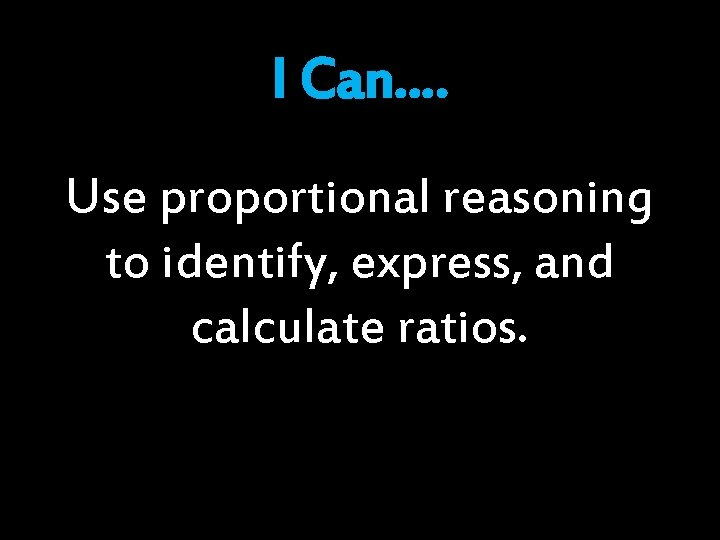I Can…. Use proportional reasoning to identify, express, and calculate ratios.Station Rotation Expectations 1. Read each task’s directions carefully, before beginning. 2. Work as a team. 3. Stay focused, productive, and on task. 4. Stay in your station area, at all times. 5. Use your resources, as needed. 6. Ask 3 before me. 7. Manage your time, you will have 25 minutes for each rotation. 8. Freeze when the timer ends and await further instructions. 9. Return all materials to the bucket before moving to the next.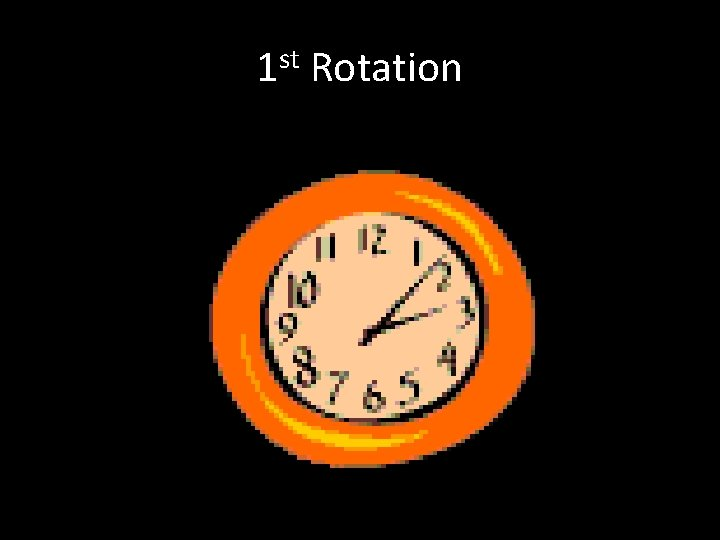1 st Rotation2 nd Rotation3 rd Rotation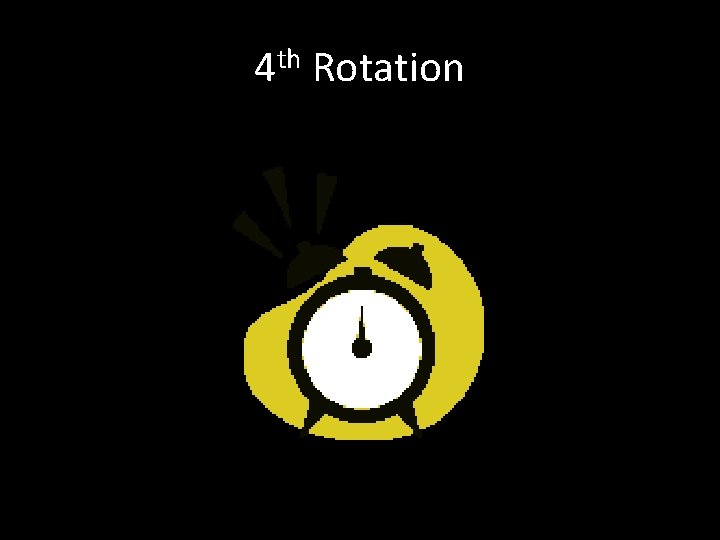4 th RotationComputer Station • http: //learnzillion. com/lessons/842 -define-unitrate-using-double-number-line • http: //learnzillion. com/lessons/613 -solve-rateproblems-using-double-number-lines • http: //learnzillion. com/lessons/612 -solve-formissing-values-in-rate-problems-using-a-table (6 minutes per video)Wrap it Up • Review • Questions • Exit Tickets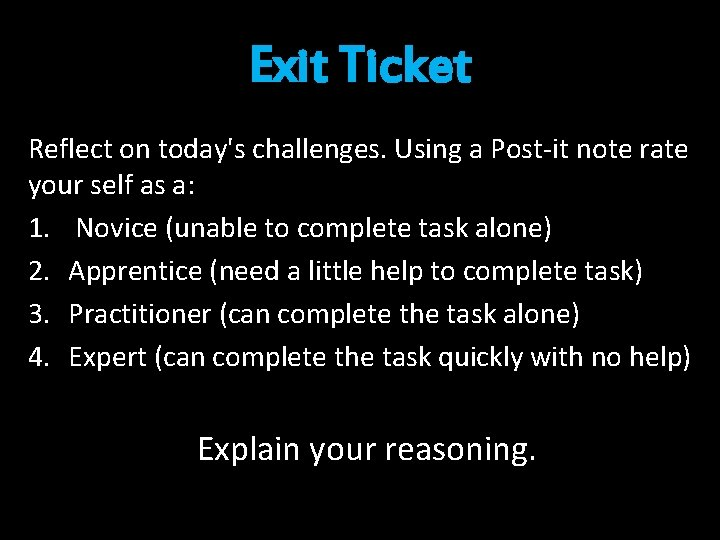Exit Ticket Reflect on today's challenges. Using a Post-it note rate your self as a: 1. Novice (unable to complete task alone) 2. Apprentice (need a little help to complete task) 3. Practitioner (can complete the task alone) 4. Expert (can complete the task quickly with no help) Explain your reasoning.Day 4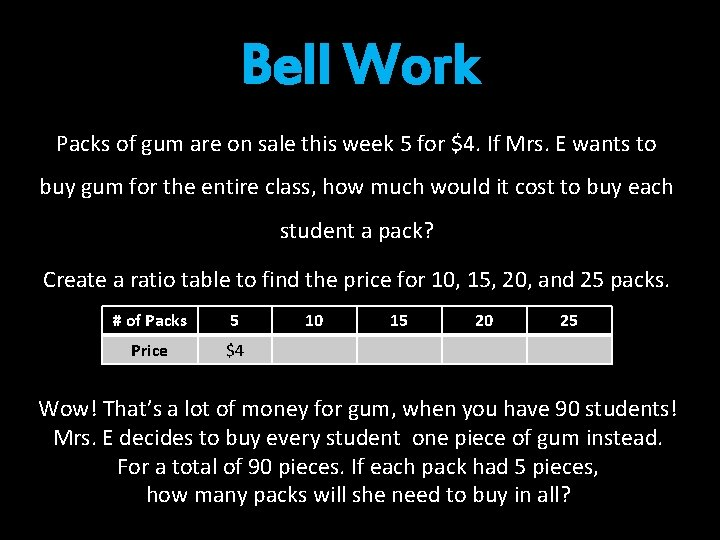Bell Work Packs of gum are on sale this week 5 for \$4. If Mrs. E wants to buy gum for the entire class, how much would it cost to buy each student a pack? Create a ratio table to find the price for 10, 15, 20, and 25 packs. # of Packs 5 Price \$4 10 15 20 25 Wow! That’s a lot of money for gum, when you have 90 students! Mrs. E decides to buy every student one piece of gum instead. For a total of 90 pieces. If each pack had 5 pieces, how many packs will she need to buy in all?Vocabulary Equivalent- Equal or the same. Fraction- A comparison of two numbers by division. A ratio that compares a part to the whole or set. Percentage- A ratio used to compare parts per 100. Represents a part to whole relationship. Proportion- An equation composed of two equivalent ratios. Rate- A ratio used to compare quantities with different units of measure. Rate of Change- Describes how one quantity changes in relation to another quantity. Ratio- A comparison of two or more quantities. Ratio Table- A table composed of equivalent ratios. Scaling- To increase or decrease a ratio, using proportional reasoning. Unit Rate- A rate decreased to a comparison of 1.Homework Check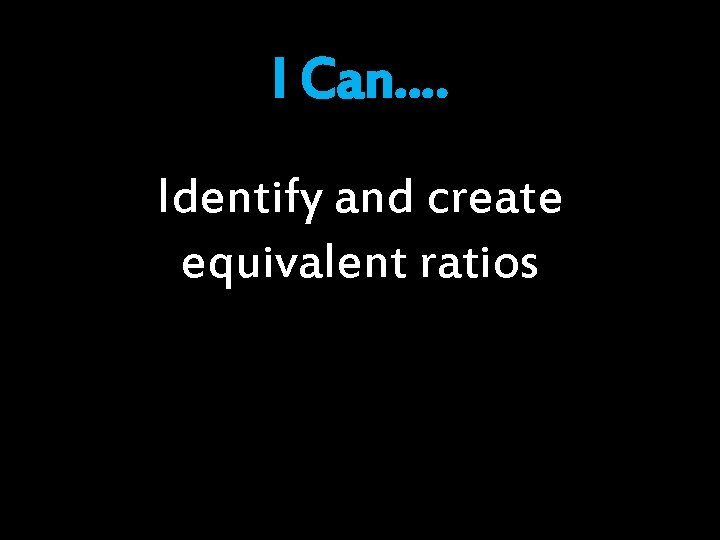I Can…. Identify and create equivalent ratiosEquivalent Ratios Essential Understanding: • • Equivalent ratios are ratios that express the same proportional relationship. You can determine if two ratios are equivalent by checking their proportionality. This is done by…. I. Calculating their unit rates- if two ratios are equivalent they will have the same unit rate. , when written in the same order. II. Determining if they have equivalent scale factors- if two ratio are equivalent, the scale factors will be equivalent. III. Calculating their cross products- If two ratios are equivalent their cross products will equivalent. Equivalent ratios can be calculated by scaling them up or down (multiplying or dividing each quantity by the same amount). You can use models when organizing and generating equivalent ratios. I. Ratio Tables a. Addition and subtraction rules a. Scaling II. Double Number Lines III. Tape Diagrams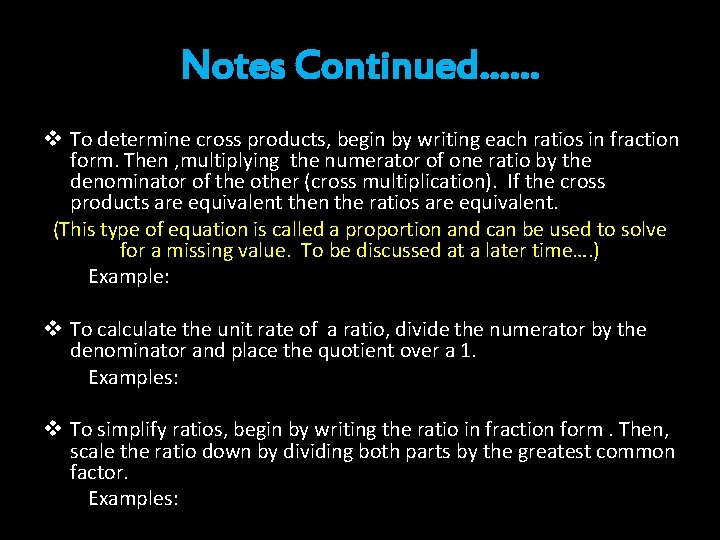Notes Continued…… v To determine cross products, begin by writing each ratios in fraction form. Then , multiplying the numerator of one ratio by the denominator of the other (cross multiplication). If the cross products are equivalent then the ratios are equivalent. (This type of equation is called a proportion and can be used to solve for a missing value. To be discussed at a later time…. ) Example: v To calculate the unit rate of a ratio, divide the numerator by the denominator and place the quotient over a 1. Examples: v To simplify ratios, begin by writing the ratio in fraction form. Then, scale the ratio down by dividing both parts by the greatest common factor. Examples:Wrap it Up • Review • Questions • Exit TicketsExit Ticket Define the following terms using your own words. 1. Ratio 2. Rate 1. Unit Rate 2. Proportional-Day 5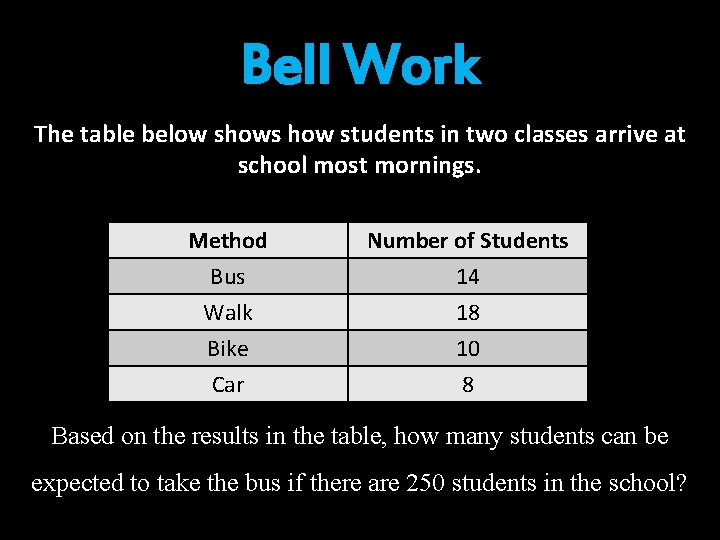Bell Work The table below shows how students in two classes arrive at school most mornings. Method Bus Walk Bike Number of Students 14 18 10 Car 8 Based on the results in the table, how many students can be expected to take the bus if there are 250 students in the school?Vocabulary Equivalent- Equal or the same. Fraction- A comparison of two numbers by division. A ratio that compares a part to the whole or set. Percentage- A ratio used to compare parts per 100. Represents a part to whole relationship. Proportion- An equation composed of two equivalent ratios. Rate- A ratio used to compare quantities with different units of measure. Rate of Change- Describes how one quantity changes in relation to another quantity. Ratio- A comparison of two or more quantities. Ratio Table- A table composed of equivalent ratios. Scaling- To increase or decrease a ratio, using proportional reasoning. Unit Rate- A rate decreased to a comparison of 1.Homework CheckPop Quiz Clear you desk of everything except for a pencil and a blank piece of paper.I Can…. Create a scale model.Lego Creation Station Clear your deskWrap it Up • Review • Questions • Exit TicketsExit Ticket Create a KWL for Chapter 3Watch This • http: //learnzillion. com/lessons/840 -createunit-rate-using-diagram (5 Minutes) • http: //learnzillion. com/lessons/841 -create-unitrate-using-tape-diagram (5 minutes)Watch This • http: //learnzillion. com/lessons/839 understand-rates-as-a-type-of-ratio (6 minutes) • http: //learnzillion. com/lessons/614 -solve-rateproblems-using-multiplicative-reasoning (7 minutes)Watch This • http: //learnzillion. com/lessons/606 -createequivalent-ratios • http: //learnzillion. com/lessons/607 -simplifyratios-using-common-factorsWatch This • http: //learnzillion. com/lessons/587 -solveratio-problems-using-tables-andmultiplication • http: //learnzillion. com/lessons/608 -solvemissing-values-in-ratio-problems-using-a-tableWatch This • http: //learnzillion. com/lessons/615 -graphingrate-problems-using-a-table (7 minutes)